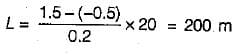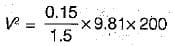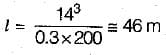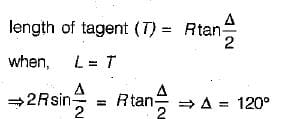# Test: Tacheometric, Curve & Hydrographic Surveying - 2

## 7 Questions MCQ Test Topicwise Question Bank for Civil Engineering | Test: Tacheometric, Curve & Hydrographic Surveying - 2

Description
Attempt Test: Tacheometric, Curve & Hydrographic Surveying - 2 | 7 questions in 20 minutes | Mock test for Civil Engineering (CE) preparation | Free important questions MCQ to study Topicwise Question Bank for Civil Engineering for Civil Engineering (CE) Exam | Download free PDF with solutions
QUESTION: 1

### If the focal length of the object glass is 25 cm and the distance from object glass to the trunnion axis is 15 cm, the additive constant is

Solution:

Additive constant = f + d = 0.25 + 0.15 = 0.40

QUESTION: 2

### If the spacing of cross hairs in a stadia diaphragm of a tacheometer is 1.2 mm and the focal length of object glass is 24 cm, then the multiplying constant of tacheometer is

Solution:QUESTION: 3

### If an upgrade of 1.5% is followed by a downgrade of 0.5% and rate of change of grade is 0.2% per 20 m chain, then the length of vertical curve is

Solution:

Length of vertical curve,QUESTION: 4

If ‘g1 ’ and ‘g2’ are the two gradients, ‘r’ is the rate of change of grade (%) per chain, the length of the vertical curve will be

Solution:
QUESTION: 5

A transition curve is required for a circular curve of 200 m radius, the gauge being 1.5 m and maximum superelevation restricted to 15 cm. The transition is to be designed for a velocity such that no lateral pressure is imposed on the rails and rate of gain of radial acceleration is 30 cm/s2. The required length of transition curve will be

Solution:⇒  V = 14 m/s
∴QUESTION: 6

The lengths of long chord and tangents of a circular curve are equal for a deflection angle of

Solution:

The length of long chord (L) = 2RsinΔ/2 and theQUESTION: 7

The staff intercepts on a vertically held staff at a distance of 50 m and 200 m were found to be 0.49 m and 1.99 m respectively. The constants of instrument used are

Solution:

D1 = 50 = 0.49 K + C   .......(i)
D2 = 200 = 1.99 K + C   ......(ii)
On solving (i).and (ii) we get,
K = 100 and C = 1Use Code STAYHOME200 and get INR 200 additional OFF Use Coupon Code